Publicité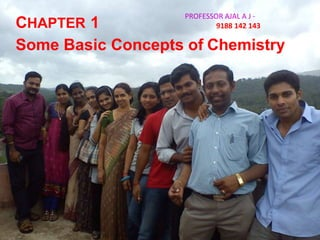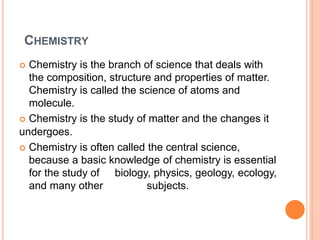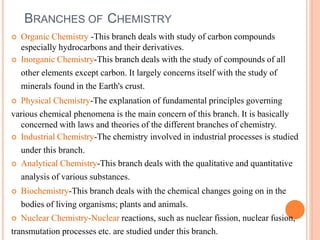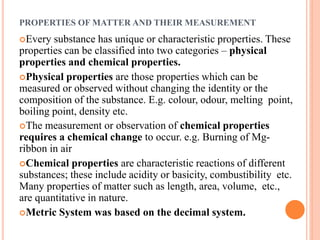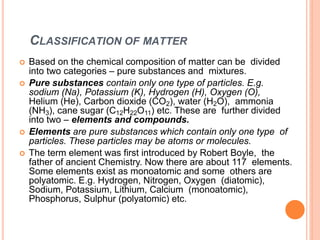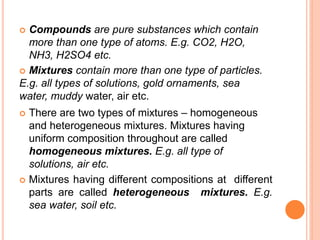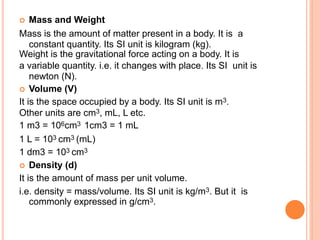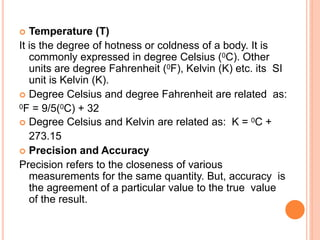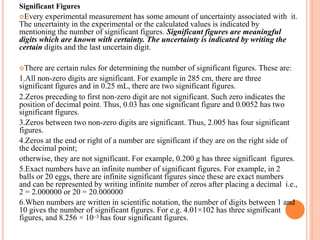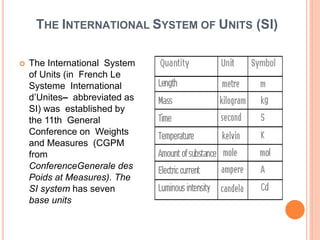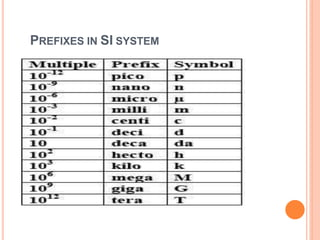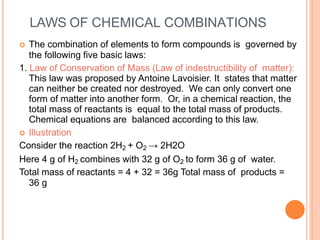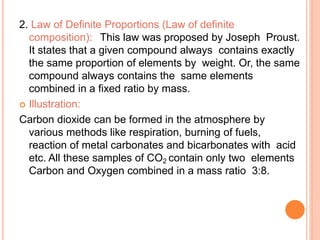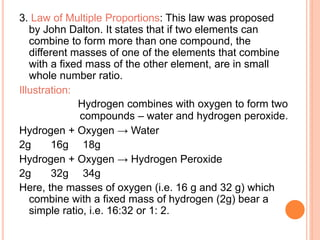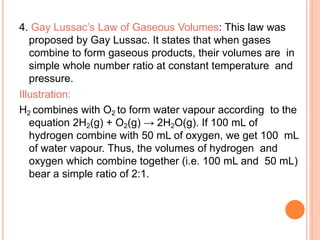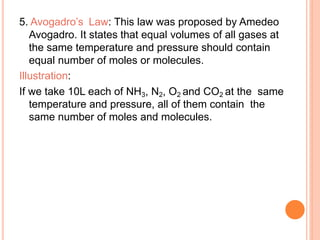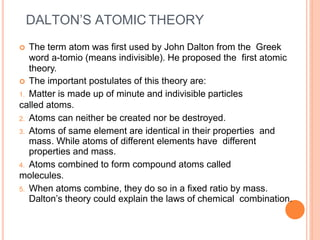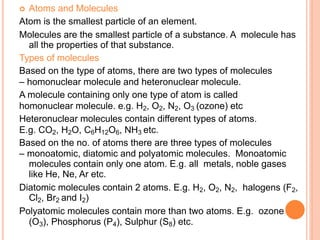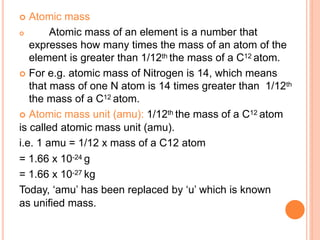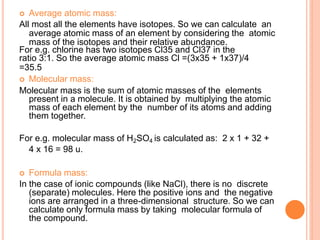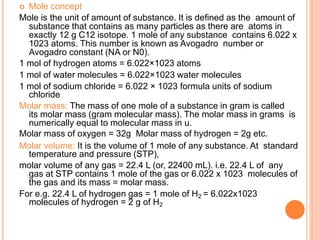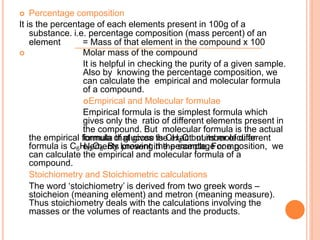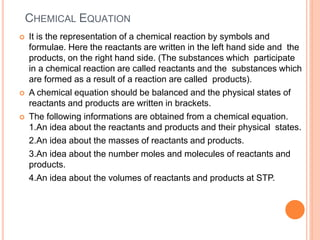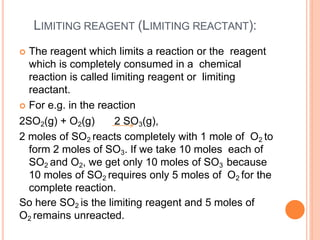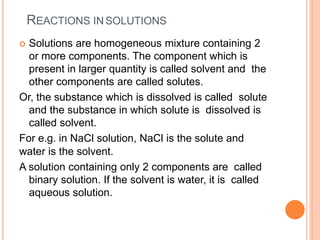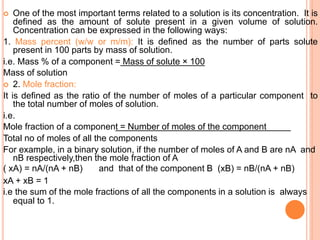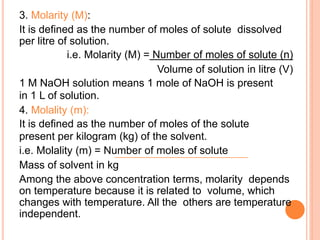1 sur 28
Publicité

### CHEMISTRY basic concepts of chemistry

1. CHAPTER 1 Some Basic Concepts of Chemistry PROFESSOR AJAL A J - 9188 142 143
2. CHEMISTRY  Chemistry is the branch of science that deals with the composition, structure and properties of matter. Chemistry is called the science of atoms and molecule.  Chemistry is the study of matter and the changes it undergoes.  Chemistry is often called the central science, because a basic knowledge of chemistry is essential for the study of biology, physics, geology, ecology, and many other subjects.
3. BRANCHES OF CHEMISTRY  Organic Chemistry -This branch deals with study of carbon compounds especially hydrocarbons and their derivatives.  Inorganic Chemistry-This branch deals with the study of compounds of all other elements except carbon. It largely concerns itself with the study of minerals found in the Earth's crust.  Physical Chemistry-The explanation of fundamental principles governing various chemical phenomena is the main concern of this branch. It is basically concerned with laws and theories of the different branches of chemistry.  Industrial Chemistry-The chemistry involved in industrial processes is studied under this branch.  Analytical Chemistry-This branch deals with the qualitative and quantitative analysis of various substances.  Biochemistry-This branch deals with the chemical changes going on in the bodies of living organisms; plants and animals.  Nuclear Chemistry-Nuclear reactions, such as nuclear fission, nuclear fusion, transmutation processes etc. are studied under this branch.
4. PROPERTIES OF MATTER AND THEIR MEASUREMENT Every substance has unique or characteristic properties. These properties can be classified into two categories – physical properties and chemical properties. Physical properties are those properties which can be measured or observed without changing the identity or the composition of the substance. E.g. colour, odour, melting point, boiling point, density etc. The measurement or observation of chemical properties requires a chemical change to occur. e.g. Burning of Mg- ribbon in air Chemical properties are characteristic reactions of different substances; these include acidity or basicity, combustibility etc. Many properties of matter such as length, area, volume, etc., are quantitative in nature. Metric System was based on the decimal system.
5. CLASSIFICATION OF MATTER  Based on the chemical composition of matter can be divided into two categories – pure substances and mixtures.  Pure substances contain only one type of particles. E.g. sodium (Na), Potassium (K), Hydrogen (H), Oxygen (O), Helium (He), Carbon dioxide (CO2), water (H2O), ammonia (NH3), cane sugar (C12H22O11) etc. These are further divided into two – elements and compounds.  Elements are pure substances which contain only one type of particles. These particles may be atoms or molecules.  The term element was first introduced by Robert Boyle, the father of ancient Chemistry. Now there are about 117 elements. Some elements exist as monoatomic and some others are polyatomic. E.g. Hydrogen, Nitrogen, Oxygen (diatomic), Sodium, Potassium, Lithium, Calcium (monoatomic), Phosphorus, Sulphur (polyatomic) etc.
6.  Compounds are pure substances which contain more than one type of atoms. E.g. CO2, H2O, NH3, H2SO4 etc.  Mixtures contain more than one type of particles. E.g. all types of solutions, gold ornaments, sea water, muddy water, air etc.  There are two types of mixtures – homogeneous and heterogeneous mixtures. Mixtures having uniform composition throughout are called homogeneous mixtures. E.g. all type of solutions, air etc.  Mixtures having different compositions at different parts are called heterogeneous mixtures. E.g. sea water, soil etc.
7.  Mass and Weight Mass is the amount of matter present in a body. It is a constant quantity. Its SI unit is kilogram (kg). Weight is the gravitational force acting on a body. It is a variable quantity. i.e. it changes with place. Its SI unit is newton (N).  Volume (V) It is the space occupied by a body. Its SI unit is m3. Other units are cm3, mL, L etc. 1 m3 = 106cm3 1cm3 = 1 mL 1 L = 103 cm3 (mL) 1 dm3 = 103 cm3  Density (d) It is the amount of mass per unit volume. i.e. density = mass/volume. Its SI unit is kg/m3. But it is commonly expressed in g/cm3.
8.  Temperature (T) It is the degree of hotness or coldness of a body. It is commonly expressed in degree Celsius (0C). Other units are degree Fahrenheit (0F), Kelvin (K) etc. its SI unit is Kelvin (K).  Degree Celsius and degree Fahrenheit are related as: 0F = 9/5(0C) + 32  Degree Celsius and Kelvin are related as: K = 0C + 273.15  Precision and Accuracy Precision refers to the closeness of various measurements for the same quantity. But, accuracy is the agreement of a particular value to the true value of the result.
9. Significant Figures Every experimental measurement has some amount of uncertainty associated with it. The uncertainty in the experimental or the calculated values is indicated by mentioning the number of significant figures. Significant figures are meaningful digits which are known with certainty. The uncertainty is indicated by writing the certain digits and the last uncertain digit. There are certain rules for determining the number of significant figures. These are: 1.All non-zero digits are significant. For example in 285 cm, there are three significant figures and in 0.25 mL, there are two significant figures. 2.Zeros preceding to first non-zero digit are not significant. Such zero indicates the position of decimal point. Thus, 0.03 has one significant figure and 0.0052 has two significant figures. 3.Zeros between two non-zero digits are significant. Thus, 2.005 has four significant figures. 4.Zeros at the end or right of a number are significant if they are on the right side of the decimal point; otherwise, they are not significant. For example, 0.200 g has three significant figures. 5.Exact numbers have an infinite number of significant figures. For example, in 2 balls or 20 eggs, there are infinite significant figures since these are exact numbers and can be represented by writing infinite number of zeros after placing a decimal i.e., 2 = 2.000000 or 20 = 20.000000 6.When numbers are written in scientific notation, the number of digits between 1 and 10 gives the number of significant figures. For e.g. 4.01×102 has three significant figures, and 8.256 × 10–3 has four significant figures.
10. THE INTERNATIONAL SYSTEM OF UNITS (SI)  The International System of Units (in French Le Systeme International d’Unites– abbreviated as SI) was established by the 11th General Conference on Weights and Measures (CGPM from ConferenceGenerale des Poids at Measures). The SI system has seven base units
11. PREFIXES IN SI SYSTEM
12. LAWS OF CHEMICAL COMBINATIONS  The combination of elements to form compounds is governed by the following five basic laws: 1. Law of Conservation of Mass (Law of indestructibility of matter): This law was proposed by Antoine Lavoisier. It states that matter can neither be created nor destroyed. We can only convert one form of matter into another form. Or, in a chemical reaction, the total mass of reactants is equal to the total mass of products. Chemical equations are balanced according to this law.  Illustration Consider the reaction 2H2 + O2 → 2H2O Here 4 g of H2 combines with 32 g of O2 to form 36 g of water. Total mass of reactants = 4 + 32 = 36g Total mass of products = 36 g
13. 2. Law of Definite Proportions (Law of definite composition): This law was proposed by Joseph Proust. It states that a given compound always contains exactly the same proportion of elements by weight. Or, the same compound always contains the same elements combined in a fixed ratio by mass.  Illustration: Carbon dioxide can be formed in the atmosphere by various methods like respiration, burning of fuels, reaction of metal carbonates and bicarbonates with acid etc. All these samples of CO2 contain only two elements Carbon and Oxygen combined in a mass ratio 3:8.
14. 3. Law of Multiple Proportions: This law was proposed by John Dalton. It states that if two elements can combine to form more than one compound, the different masses of one of the elements that combine with a fixed mass of the other element, are in small whole number ratio. Illustration: Hydrogen combines with oxygen to form two compounds – water and hydrogen peroxide. Hydrogen + Oxygen → Water 2g 16g 18g Hydrogen + Oxygen → Hydrogen Peroxide 2g 32g 34g Here, the masses of oxygen (i.e. 16 g and 32 g) which combine with a fixed mass of hydrogen (2g) bear a simple ratio, i.e. 16:32 or 1: 2.
15. 4. Gay Lussac’s Law of Gaseous Volumes: This law was proposed by Gay Lussac. It states that when gases combine to form gaseous products, their volumes are in simple whole number ratio at constant temperature and pressure. Illustration: H2 combines with O2 to form water vapour according to the equation 2H2(g) + O2(g) → 2H2O(g). If 100 mL of hydrogen combine with 50 mL of oxygen, we get 100 mL of water vapour. Thus, the volumes of hydrogen and oxygen which combine together (i.e. 100 mL and 50 mL) bear a simple ratio of 2:1.
16. 5. Avogadro’s Law: This law was proposed by Amedeo Avogadro. It states that equal volumes of all gases at the same temperature and pressure should contain equal number of moles or molecules. Illustration: If we take 10L each of NH3, N2, O2 and CO2 at the same temperature and pressure, all of them contain the same number of moles and molecules.
17. DALTON’S ATOMIC THEORY  The term atom was first used by John Dalton from the Greek word a-tomio (means indivisible). He proposed the first atomic theory.  The important postulates of this theory are: 1. Matter is made up of minute and indivisible particles called atoms. 2. Atoms can neither be created nor be destroyed. 3. Atoms of same element are identical in their properties and mass. While atoms of different elements have different properties and mass. 4. Atoms combined to form compound atoms called molecules. 5. When atoms combine, they do so in a fixed ratio by mass. Dalton’s theory could explain the laws of chemical combination.
18.  Atoms and Molecules Atom is the smallest particle of an element. Molecules are the smallest particle of a substance. A molecule has all the properties of that substance. Types of molecules Based on the type of atoms, there are two types of molecules – homonuclear molecule and heteronuclear molecule. A molecule containing only one type of atom is called homonuclear molecule. e.g. H2, O2, N2, O3 (ozone) etc Heteronuclear molecules contain different types of atoms. E.g. CO2, H2O, C6H12O6, NH3 etc. Based on the no. of atoms there are three types of molecules – monoatomic, diatomic and polyatomic molecules. Monoatomic molecules contain only one atom. E.g. all metals, noble gases like He, Ne, Ar etc. Diatomic molecules contain 2 atoms. E.g. H2, O2, N2, halogens (F2, Cl2, Br2 and I2) Polyatomic molecules contain more than two atoms. E.g. ozone (O3), Phosphorus (P4), Sulphur (S8) etc.
19.  Atomic mass  Atomic mass of an element is a number that expresses how many times the mass of an atom of the element is greater than 1/12th the mass of a C12 atom.  For e.g. atomic mass of Nitrogen is 14, which means that mass of one N atom is 14 times greater than 1/12th the mass of a C12 atom.  Atomic mass unit (amu): 1/12th the mass of a C12 atom is called atomic mass unit (amu). i.e. 1 amu = 1/12 x mass of a C12 atom = 1.66 x 10-24 g = 1.66 x 10-27 kg Today, ‘amu’ has been replaced by ‘u’ which is known as unified mass.
20.  Average atomic mass: All most all the elements have isotopes. So we can calculate an average atomic mass of an element by considering the atomic mass of the isotopes and their relative abundance. For e.g. chlorine has two isotopes Cl35 and Cl37 in the ratio 3:1. So the average atomic mass Cl =(3x35 + 1x37)/4 =35.5  Molecular mass: Molecular mass is the sum of atomic masses of the elements present in a molecule. It is obtained by multiplying the atomic mass of each element by the number of its atoms and adding them together. For e.g. molecular mass of H2SO4 is calculated as: 2 x 1 + 32 + 4 x 16 = 98 u.  Formula mass: In the case of ionic compounds (like NaCl), there is no discrete (separate) molecules. Here the positive ions and the negative ions are arranged in a three-dimensional structure. So we can calculate only formula mass by taking molecular formula of the compound.
21.  Mole concept Mole is the unit of amount of substance. It is defined as the amount of substance that contains as many particles as there are atoms in exactly 12 g C12 isotope. 1 mole of any substance contains 6.022 x 1023 atoms. This number is known as Avogadro number or Avogadro constant (NA or N0). 1 mol of hydrogen atoms = 6.022×1023 atoms 1 mol of water molecules = 6.022×1023 water molecules 1 mol of sodium chloride = 6.022 × 1023 formula units of sodium chloride Molar mass: The mass of one mole of a substance in gram is called its molar mass (gram molecular mass). The molar mass in grams is numerically equal to molecular mass in u. Molar mass of oxygen = 32g Molar mass of hydrogen = 2g etc. Molar volume: It is the volume of 1 mole of any substance. At standard temperature and pressure (STP), molar volume of any gas = 22.4 L (or, 22400 mL). i.e. 22.4 L of any gas at STP contains 1 mole of the gas or 6.022 x 1023 molecules of the gas and its mass = molar mass. For e.g. 22.4 L of hydrogen gas = 1 mole of H2 = 6.022x1023 molecules of hydrogen = 2 g of H2
22.  Percentage composition It is the percentage of each elements present in 100g of a substance. i.e. percentage composition (mass percent) of an element = Mass of that element in the compound x 100  Molar mass of the compound It is helpful in checking the purity of a given sample. Also by knowing the percentage composition, we can calculate the empirical and molecular formula of a compound. Empirical and Molecular formulae Empirical formula is the simplest formula which gives only the ratio of different elements present in the compound. But molecular formula is the actual formula that gives the exact number of different elements present in the sample. For e.g. the empirical formula of glucose is CH2O but its molecular formula is C6H12O6. By knowing the percentage composition, we can calculate the empirical and molecular formula of a compound. Stoichiometry and Stoichiometric calculations The word ‘stoichiometry’ is derived from two greek words – stoicheion (meaning element) and metron (meaning measure). Thus stoichiometry deals with the calculations involving the masses or the volumes of reactants and the products.
23. CHEMICAL EQUATION  It is the representation of a chemical reaction by symbols and formulae. Here the reactants are written in the left hand side and the products, on the right hand side. (The substances which participate in a chemical reaction are called reactants and the substances which are formed as a result of a reaction are called products).  A chemical equation should be balanced and the physical states of reactants and products are written in brackets.  The following informations are obtained from a chemical equation. 1.An idea about the reactants and products and their physical states. 2.An idea about the masses of reactants and products. 3.An idea about the number moles and molecules of reactants and products. 4.An idea about the volumes of reactants and products at STP.
24. LIMITING REAGENT (LIMITING REACTANT):  The reagent which limits a reaction or the reagent which is completely consumed in a chemical reaction is called limiting reagent or limiting reactant.  For e.g. in the reaction 2SO2(g) + O2(g) 2 SO3(g), 2 moles of SO2 reacts completely with 1 mole of O2 to form 2 moles of SO3. If we take 10 moles each of SO2 and O2, we get only 10 moles of SO3 because 10 moles of SO2 requires only 5 moles of O2 for the complete reaction. So here SO2 is the limiting reagent and 5 moles of O2 remains unreacted.
25. REACTIONS INSOLUTIONS  Solutions are homogeneous mixture containing 2 or more components. The component which is present in larger quantity is called solvent and the other components are called solutes. Or, the substance which is dissolved is called solute and the substance in which solute is dissolved is called solvent. For e.g. in NaCl solution, NaCl is the solute and water is the solvent. A solution containing only 2 components are called binary solution. If the solvent is water, it is called aqueous solution.
26.  One of the most important terms related to a solution is its concentration. It is defined as the amount of solute present in a given volume of solution. Concentration can be expressed in the following ways: 1. Mass percent (w/w or m/m): It is defined as the number of parts solute present in 100 parts by mass of solution. i.e. Mass % of a component = Mass of solute × 100 Mass of solution  2. Mole fraction: It is defined as the ratio of the number of moles of a particular component to the total number of moles of solution. i.e. Mole fraction of a component = Number of moles of the component Total no of moles of all the components For example, in a binary solution, if the number of moles of A and B are nA and nB respectively,then the mole fraction of A ( xA) = nA/(nA + nB) and that of the component B (xB) = nB/(nA + nB) xA + xB = 1 i.e the sum of the mole fractions of all the components in a solution is always equal to 1.
27. 3. Molarity (M): It is defined as the number of moles of solute dissolved per litre of solution. i.e. Molarity (M) = Number of moles of solute (n) Volume of solution in litre (V) 1 M NaOH solution means 1 mole of NaOH is present in 1 L of solution. 4. Molality (m): It is defined as the number of moles of the solute present per kilogram (kg) of the solvent. i.e. Molality (m) = Number of moles of solute Mass of solvent in kg Among the above concentration terms, molarity depends on temperature because it is related to volume, which changes with temperature. All the others are temperature independent.
Publicité